# Implementation of the PATH Statement

Ewout Steyerberg
e.w.steyerberg@lumc.nl
ORCID

Evidence is derived from groups while most medical decisions are made for individual patients
(Kent et al, PATH statement)

Heterogeneity of treatment effect (HTE) refers to the nonrandom variation in the magnitude of the absolute treatment effect (treatment benefit) across individual patients. The recent PATH (Predictive Approaches to Treatment effect Heterogeneity) Statement outlines principles, criteria, and key considerations for applying predictive approaches to clinical trials to provide patient-centered evidence in support of decision making. The focus of PATH is on modeling of HTE across individual patients.

The PATH statement lists a number of principles and guidelines. A first principle is to establish overall treatment effect. In another blog, I summarized the arguments in favor of covariate adjustment as the primary analysis of a RCT. Illustration was in the GUSTO-I trial. Here I continue that illustration, also following the blog by Frank Harrell on examining HTE.

## Illustration in the GUSTO-I trial

Let’s analyze the data from 30,510 patients with an acute myocardial infarction as included in the GUSTO-I trial. In GUSTO-I, 10,348 patients were randomized to receive tissue plasminogen activator (tPA), while 20,162 were randomzied to Streptokinase (SK) and had 30-day mortality status known.

Input object size:	 4042624 bytes;	 29 variables	 30510 observations
Kept variables	day30,tx,age,Killip,sysbp,pulse,pmi,miloc,sex
New object size:	1350344 bytes;	9 variables	30510 observations


gusto

9 Variables   30510 Observations

day30

nmissingdistinctInfoSumMeanGmd
30510020.19521280.069750.1298

sex: Sex
nmissingdistinct
3051002
 Value        male female
Frequency   22795   7715
Proportion  0.747  0.253


Killip: Killip Class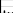nmissingdistinct
3051004
 Value          I    II   III    IV
Frequency  26007  3857   417   229
Proportion 0.852 0.126 0.014 0.008


age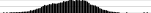nmissingdistinctInfoMeanGmd.05.10.25.50.75.90.95
3051005342160.9113.5840.9244.7352.1161.5869.8476.1979.42
lowest : 19.0 20.8 21.0 21.0 21.4 , highest: 91.9 92.3 96.5 108.0 110.0
pulse: Heart Rate beats/min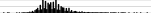nmissingdistinctInfoMeanGmd.05.10.25.50.75.90.95
3051001570.99975.3819.5505562738698107
lowest : 0 1 6 9 20 , highest: 191 200 205 210 220
sysbp: Systolic Blood Pressure mmHg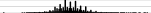nmissingdistinctInfoMeanGmd.05.10.25.50.75.90.95
3051001960.99912926.5892.0100.0112.0129.5144.0160.0170.0
lowest : 0 36 40 43 46 , highest: 266 274 275 276 280
miloc: MI Location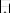nmissingdistinct
3051003
 Value      Inferior    Other Anterior
Frequency     17582     1062    11866
Proportion    0.576    0.035    0.389


pmi: Previous MI
nmissingdistinct
3051002
 Value         no   yes
Frequency  25452  5058
Proportion 0.834 0.166


tx: Tx in 3 groups
nmissingdistinct
3051002
 Value         SK   tPA
Frequency  20162 10348
Proportion 0.661 0.339


### Overall treatment effect

The primary outcome was 30-day mortality. Among the tPA group, the 30-day mortality was 653/10,348 = 6.3% vs 1475/20,162 = 7.3% in the SK group. This an absolute difference of 1.0%, or an odds ratio of 0.85 [0.78-0.94].

SK
(N=20162)
tPA
(N=10348)
Overall
(N=30510)
as.factor(day30)
018687 (92.7%)9695 (93.7%)28382 (93.0%)
11475 (7.3%)653 (6.3%)2128 (7.0%)
Odds RatioLower CIUpper CI
0.8530.7760.939
Absolute differenceLower CIUpper CI
0.010.0040.016

The unadjusted odds ratio of 0.853 is a marginal estimate. As explained in the other blog, a lot can be said in favor of conditional estimates, where we adjust for prognostically important baseline characteristics.
In line with Califf 1997 and Steyerberg 2000, we consider a prediction model with 6 baseline covariates, including age and Killip class (a measure for ventricular function). Pulse rate is modeled using a linear spline with a knot at 50 beats/minute.

Logistic Regression Model

 lrm(formula = day30 ~ tpa + age + Killip + pmin(sysbp, 120) +
lsp(pulse, 50) + pmi + miloc, data = gusto, x = T, maxit = 99)

Model Likelihood
Ratio Test
Discrimination
Indexes
Rank Discrim.
Indexes
Obs 30510LR χ2 2991.95R2 0.235C 0.815
0 28382d.f. 11g 1.381Dxy 0.631
1 2128Pr(>χ2) <0.0001gr 3.979γ 0.631
max |∂log L/∂β| 6×10-10gp 0.080τa 0.082
Brier 0.056
βS.E.Wald ZPr(>|Z|)
Intercept -3.0203 0.7973-3.790.0002
tpa -0.2080 0.0529-3.93<0.0001
age  0.0769 0.002531.28<0.0001
Killip=II  0.6137 0.058910.42<0.0001
Killip=III  1.1610 0.12149.57<0.0001
Killip=IV  1.9213 0.161811.87<0.0001
sysbp -0.0392 0.0019-20.33<0.0001
pulse -0.0242 0.0159-1.520.1282
pulse'  0.0433 0.01622.670.0075
pmi=yes  0.4472 0.05627.96<0.0001
miloc=Other  0.2863 0.13472.130.0335
miloc=Anterior  0.5432 0.051110.62<0.0001

So, we note that the adjusted regression coefficient for tPA was -0.208. The adjusted OR = 0.812.
Let’s check for statistical interaction with

1. Individual covariates
2. The linear predictor
## check for interaction
g <- lrm(day30 ~  tx * (age + Killip + pmin(sysbp, 120) + lsp(pulse, 50) + pmi + miloc),
data=gusto, maxit=100)
print(anova(g)) # tx interactions: 10 df, p=0.5720; based on LR test

 χ2 d.f. P Wald Statistics for day30 tx (Factor+Higher Order Factors) 23.48 11 0.0151 All Interactions 8.53 10 0.5772 age (Factor+Higher Order Factors) 981.65 2 <0.0001 All Interactions 1.60 1 0.2065 Killip (Factor+Higher Order Factors) 281.44 6 <0.0001 All Interactions 1.37 3 0.7131 sysbp (Factor+Higher Order Factors) 412.30 2 <0.0001 All Interactions 0.11 1 0.7369 pulse (Factor+Higher Order Factors) 235.92 4 <0.0001 All Interactions 4.22 2 0.1214 Nonlinear (Factor+Higher Order Factors) 7.37 2 0.0251 pmi (Factor+Higher Order Factors) 64.23 2 <0.0001 All Interactions 0.05 1 0.8233 miloc (Factor+Higher Order Factors) 115.23 4 <0.0001 All Interactions 2.99 2 0.2239 tx × age (Factor+Higher Order Factors) 1.60 1 0.2065 tx × Killip (Factor+Higher Order Factors) 1.37 3 0.7131 tx × sysbp (Factor+Higher Order Factors) 0.11 1 0.7369 tx × pulse (Factor+Higher Order Factors) 4.22 2 0.1214 Nonlinear 0.08 1 0.7784 Nonlinear Interaction : f(A,B) vs. AB 0.08 1 0.7784 tx × pmi (Factor+Higher Order Factors) 0.05 1 0.8233 tx × miloc (Factor+Higher Order Factors) 2.99 2 0.2239 TOTAL NONLINEAR 7.37 2 0.0251 TOTAL INTERACTION 8.53 10 0.5772 TOTAL NONLINEAR + INTERACTION 15.57 11 0.1579 TOTAL 2343.20 21 <0.0001
# 2. PATH statement: linear interaction with linear predictor; baseline risk, so tx=ref, SK
lp.no.tx <- f$coefficients + 0 * f$coefficients + f$x[,-1] %*% f$coefficients[-(1:2)]
gusto$lp <- as.vector(lp.no.tx) # add lp to data frame h <- lrm(day30 ~ tx * lp, data=gusto) print(anova(h)) # tx interaction: 1 df, p=0.35; based on Wald statistics   χ2 d.f. P Wald Statistics for day30 tx (Factor+Higher Order Factors) 16.24 2 0.0003 All Interactions 0.86 1 0.3526 lp (Factor+Higher Order Factors) 2338.37 2 <0.0001 All Interactions 0.86 1 0.3526 tx × lp (Factor+Higher Order Factors) 0.86 1 0.3526 TOTAL 2342.20 3 <0.0001 So: 1. The overall test for interaction with the individual covariates is far from statistically significant (p>0.5). 2. Similarly, the test for interaction with the linear predictor is far from statistically significant (p>0.3). #### Conclusion: no interaction needed We conclude that we may proceed by ignoring any interactions. We have no evidence against the assumption that the overall effect of treatment is applicable to all patients. The patients vary widely in risk, as can easily be seen in the histogram below.We note that many patients have baseline risks (tPA==0) below 5%. Obviously their maximum benefit is bounded by this risk estimate, even if tPA, hypothetically, would reduce the risk to Null. ## PATH principle: perform risk-based subgrouping Fig 3 of the PATH Statement starts with: Reporting RCT results stratified by a risk model is encouraged when overall trial results are positive to better understand the distribution of effects across the trial population. How do we provide such reporting of RCT results? We can provide estimates of relative effects and absolute benefit 1. By group (e.g. quarters defined by quartiles) 2. By baseline risk (continuous, as in the histogram above) ### 1a. Relative effects of treatment by risk-group The checks for interaction were far from statistically significant in GUSTO-I. We can further illustrate the relative effects in a forest plot. Many reports from RCTs include forest plots that show relative effects by single variables, such as men vs women; young vs old age; disease subtype; etc. The PATH statement encourages reporting by risk-based subgroup. How can such reporting be done? Let’s do some data processing to make a better forest plot. #### A PATH compatible forest plot Let’s expand the standard forest plot for subgroup effects with risk-based subgroups. Below subgroup effects are defined for 4 risk-based groups using cut2(lp.no.tx, g=4), and for 3 classical subgroups (by sex, age, type of infarction). ## quantiles, suggest to use quarters groups <- cut2(lp.no.tx, g=4) group0 <- groups[gusto$tpa==0]  # SK gropup
group1 <- groups[gusto$tpa==1] # tPA group rate0 <- prop.table(table(group0, gusto$day30[gusto$tpa==0]),1 )[,2] rate1 <- prop.table(table(group1, gusto$day30[gusto$tpa==1]),1 )[,2] ratediff <- rate0-rate1 # benefit of tPA by group # Make a data frame for the results data.subgroups <- as.data.frame(matrix(nrow=(4+6+1), ncol=10)) colnames(data.subgroups) <- c("tevent", "tnoevent", "cevent", "cnoevent", "name", "type", "tn", "pt", "cn", "pc") data.subgroups[11,1:4] <- table(gusto$tpa,gusto$day30)[4:1] # overall results # define event and non-event numbers events1 <- table(group0, gusto$day30[gusto$tpa==0])[,2] nevents1 <- table(group0, gusto$day30[gusto$tpa==0])[,1] events2 <- table(group1, gusto$day30[gusto$tpa==1])[,2] nevents2 <- table(group1, gusto$day30[gusto$tpa==1])[,1] n1 <- events1 + nevents1 n2 <- events2 + nevents2 data.subgroups[10:7,1:4] <- cbind(events2,nevents2,events1,nevents1)  Data for classic subgroups are managed below: # Use table to get the summary of cell numbers, by subgroup # SEX data.subgroups[5,1:4] <- table(1-gusto$day30,1-gusto$tpa, gusto$sex)[1:4]
data.subgroups[6,1:4] <- table(1-gusto$day30,1-gusto$tpa, gusto$sex)[5:8] # AGE data.subgroups[3,1:4] <- table(1-gusto$day30,1-gusto$tpa, gusto$age>=75)[1:4]
data.subgroups[4,1:4] <- table(1-gusto$day30,1-gusto$tpa, gusto$age>=75)[5:8] # ANT data.subgroups[1,1:4] <- table(1-gusto$day30,1-gusto$tpa, gusto$miloc=="Anterior")[1:4]
data.subgroups[2,1:4] <- table(1-gusto$day30,1-gusto$tpa, gusto$miloc=="Anterior")[5:8] # Names data.subgroups[11,5] <- "Overall" data.subgroups[10:7,5] <- paste("Quarter",1:4, sep=" ") data.subgroups[5:6,5] <- c("Male sex","Female sex") data.subgroups[3:4,5] <- c("Age <75","Age>=75") data.subgroups[1:2,5] <- c("Other MI","Anterior") # Type of subgroup data.subgroups[11,6] <- "" data.subgroups[10:7,6] <- c(rep("Risk-based subgroups", length(ratediff))) data.subgroups[1:6,6] <- c(rep("Location",2), rep("Age",2), rep("Sex",2)) data.subgroups[,7] <- data.subgroups[,1] + data.subgroups[,2] data.subgroups[,8] <- paste(round(100*data.subgroups[,1] / data.subgroups[,7] , 1),"%", sep="") data.subgroups[,9] <- data.subgroups[,3] + data.subgroups[,4] data.subgroups[,10] <- paste(round(100*data.subgroups[,3] / data.subgroups[,9] , 1),"%", sep="") # Show the data kable(as.data.frame((data.subgroups))) %>% kable_styling(full_width=F, position = "left")  teventtnoeventceventcnoeventnametypetnptcnpc 301602564911669Other MILocation63264.8%123185.3% 35236708267018AnteriorLocation40228.8%784410.5% 397862298116781Age <75Age90194.4%177625.5% 25610734941906Age>=75Age132919.3%240020.6% 383734288814182Male sexSex77255%150705.9% 27023535874505Female sexSex262310.3%509211.5% 455216310004009Quarter 4Risk-based subgroups261817.4%500920% 13024793004719Quarter 3Risk-based subgroups26095%50196% 4924811304967Quarter 2Risk-based subgroups25301.9%50972.6% 192572454992Quarter 1Risk-based subgroups25910.7%50370.9% 6531475969518687Overall212830.7%2838234.2% In this table tevent means #events among treated; cevent means #events among non-treated; etc Results can be plotted with metafor functions: par(mar=c(4,4,1,2)) ### fit random-effects model (use slab argument to define "study" labels) res <- rma(ai=tevent, bi=tnoevent, ci=cevent, di=cnoevent, data=data.subgroups, measure="OR", slab=name, method="ML") ### set up forest plot (with 2x2 table counts added); rows argument is used ### to specify exactly in which rows the outcomes will be plotted) forest(res, xlim=c(-8, 2.5), at=log(c(0.5, 1)), alim=c(log(0.2), log(2)), atransf=exp, ilab=cbind(data.subgroups$tn, data.subgroups$pt, data.subgroups$cn, data.subgroups$pc), ilab.xpos=c(-5,-4,-3,-2), adj=1, cex=.9, ylim=c(0, 19), rows=c(1:2, (4:5)-.5, 6:7, 10:13, 15), xlab="", mlab="", psize=.8, addfit=F) # lines(x=c(-.15, -.15), y=c(0, 17)) ## could add a reference line of the overall treatment effect text(c(-5,-4,-3,-2, 2.2), 18, c("n", "%mort", "n", "%mort", "OR [95% CI]"), font=2, adj=1, cex=.9) text(-8, 18, c("GUSTO-I trial"), font=2, adj=0, cex=.9) text(c(-4.5,-2.5), 19, c("tPA", "SK"), font=2, adj=1) # This can be improvedThis forest plot shows the unadjusted overall effect of tPA vs SK treatment; risk-based subgroup effects; and traditional one at a time subgroup effects. The latter are to be interpreted with much caution; many false-positive findings may arise. Q: What R function can assist trialists in their reporting of risk-based subgroups together with classic subgroups? We can also estimate the same subgroup effects, adjusted for baseline risk. # function for adjustment subgroup.adj <- function(data=gusto, subgroup=gusto$sex) {
coef.unadj <- by(data, subgroup, function(x)lrm.fit(y=x$day30, x=x$tpa)$coef) var.unadj <- by(data, subgroup, function(x)lrm.fit(y=x$day30, x=x$tpa)$var[2,2])
coef.adj   <- by(data, subgroup, function(x)lrm.fit(y=x$day30, x=x$tpa, offset=x$lp)$coef)
var.adj    <- by(data, subgroup, function(x)lrm.fit(y=x$day30, x=x$tpa, offset=x$lp)$var[2,2])
result} # end function

options(digits=3)


TRUE-0.159-0.2081.310.0490.0531.09

Risk-based subgroups

[-7.18,-4.01)-0.199-0.1980.9930.2750.2751.00
[-4.01,-3.20)-0.282-0.2810.9980.1690.1701.00
[-3.20,-2.36)-0.193-0.1870.9710.1080.1081.00
[-2.36, 4.70]-0.170-0.2081.2190.0630.0671.07

Classic subgroup: men vs women

male-0.183-0.2371.290.0630.0681.08
female-0.127-0.1641.290.0780.0851.10
Classic subgroup: old (>=75) vs younger age
FALSE-0.239-0.2621.100.0610.0651.06
TRUE-0.083-0.1001.210.0860.0931.08

The unadjusted and adjusted results are usually quite in line; only subtle differences is the estimates of the relative effects are noted. We might hypothesize that the unadjusted effect for women is confounded by the higher age of women (where higher age was associated with a somewhat weaker treatment effect); this was not confirmed.

Q: What R function can be developed that extends the unadjusted forest plots to provide subgroup effects, adjusted for baseline characteristics?

### 1b. Absolute benefit of treatment by risk-group

# 95% CI
CI      <- BinomDiffCI(x1 = events1, n1 = n1, x2 = events2, n2 = n2, method = "scorecc")

colnames(CI) <- c("Absolute difference", "Lower CI", "Upper CI")
rownames(CI) <- names(events1)

result <- round(CI, 3) # absolute difference with confidence interval
kable(as.data.frame(result)) %>% kable_styling(full_width=F, position = "left")

Absolute differenceLower CIUpper CI
[-7.18,-4.01)0.002-0.0030.006
[-4.01,-3.20)0.006-0.0010.013
[-3.20,-2.36)0.010-0.0010.020
[-2.36, 4.70]0.0260.0070.044

So, we see a substantial difference in absolute benefit. Low risk according to the linear predictor (lp<-2.36) implies low benefit (<1%); higher risk, higher benefit (>2%). As Frank Harrell would also emphasize, the grouping in quarters might primarily be considered for illustration. A better estimation of benefit avoids grouping, and conditions on the baseline risk.

### 2a. Relative effects of treatment by baseline risk

The checks for interaction with the linear predictor were far from statistically significant in GUSTO-I, as shown above; supporting the assumption that 1 single adjusted, relative effect applies across baseline risk.

### 2b. Absolute benefit of treatment by baseline risk

Estimation of absolute benefit can follow a parametric approach, i.e. following the no interaction, main effect model that includes baseline characteristics and a treatment effect; the model f considered above for the primary analysis of the trial.
Further down we will consider relaxations of the proportionality of effect that is assumed in this model.

# create baseline predictions: X-axis
xp <- seq(0.002,.5,by=0.001)
logxp0 <- log(xp/(1-xp))

# expected difference, if covariate adjusted model holds
p1exp <- plogis(logxp0) - plogis(logxp0+coef(f)) # proportional effect assumed

plot(x=xp, y=p1exp, type='l', lty=2, lwd=3, xlim=c(0,.35), ylim=c(-0.007,.05), col="red",
xlab="Baseline risk", ylab="Benefit by tPA", cex.lab=1.2, las=1, bty='l' )
lines(x=c(0,.5), y=c(0,0))
# distribution of predicted

points(x=rate0, y=ratediff, pch=1, cex=2, lwd=2, col="blue")
arrows(x0=rate0, x1=rate0, y0=CI[,2], y1=CI[,3], angle=90, code=3,len=.1, col="blue")

legend("topleft", lty=c(2,NA), pch=c(NA,1), lwd=c(3,2), bty='n',col=c("red", "blue"), cex=1.2,
legend=c("Expected with proportional effect",
"Grouped patients"))This plot shows the benefit by tPA treatment over SK. The red line assumes a proportional effect of treatment, which may be quite reasonable here and in many other diseases. The quarters provide for a non-parametric confirmation of the benefit across baseline risk.

### Relaxation of the proportional effect assumption

If we want to relax the proportional effect assumption, the blog by Frank Harrell on examining HTE provides an illustration with penalized logistic regression.

Another possible relaxation is by including interaction with the linear predictor. We consider linear interaction and a non-linear interaction (rcs, 2 df for non-linearity).
And we could try a more non-paramteric approach as in Califf 1997. There, loess smoothers were used for risk estimation the tPA (day30~lp, subset=tpa==1) and SK groups (day30~lp, subset=tpa==0).
Benefit was the differences between these 2 risk groups conditional on baseline risk.

h  <- lrm(day30 ~ tpa + tpa * lp, data=gusto, eps=0.005, maxit=30)
h2 <- lrm(day30 ~ tpa + rcs(lp,3)*tpa, data=gusto, eps=0.005, maxit=99)
# loess smoothing
l0 <- loess(day30 ~ lp, data=gusto, subset=tpa==0)
l1 <- loess(day30 ~ lp, data=gusto, subset=tpa==1)

# subtract predicted risks with from without tx
p1 <- plogis(Predict(h,  tpa=0, lp = logxp0)[,3]) -
plogis(Predict(h,  tpa=1, lp = logxp0)[,3])
p2 <- plogis(Predict(h2, tpa=0, lp = logxp0)[,3]) -
plogis(Predict(h2, tpa=1, lp = logxp0)[,3])
l  <- predict(l0, data.frame(lp = logxp0)) -
predict(l1, data.frame(lp = logxp0))

plot(x=xp, y=p1exp, type='l', lty=1, lwd=4, xlim=c(0,.35), ylim=c(-0.007,.05), col="red",
xlab="Baseline risk", ylab="Benefit by tPA", cex.lab=1.2, las=1, bty='l' )
# benefit with interaction terms
lines(x=xp, y=p1, type='l', lty=2, lwd=3, col="dark blue")
lines(x=xp, y=p2, type='l', lty=3, lwd=2, col="purple")
lines(x=xp, y=l,  type='l', lty=1, lwd=3, col="black")

# horizontal line
lines(x=c(0,.5), y=c(0,0))
# distribution of predicted

legend("topleft", lty=c(1,2,3,1), pch=c(NA,NA,NA,NA), lwd=c(4,3,2,3), bty='n',
col=c("red", "dark blue", "purple","black"), cex=1.2,
legend=c("Expected with proportional effect",
"Linear interaction", "Spline smoothing, 2 df",
"Loess"))This plot confirms that all estimates of benefit by baseline risk are more or less similar, with benefit clearly increasing by baseline risk. For very low baseline risk, the loess estimates are implausible.

We can also add the grouped observations by decile, as in Califf 1997. The 95% confidence intervals show that the uncertainty per risk group is huge.

Q: How many risk-based groups should be used for illustration of benefit by risk?
Default: use quartiles to define 4 quarters; perhaps 3 or only 2 groups in smaller trials?## Conclusions

The GUSTO-I trial serves well to illustrate the impact of conditioning on baseline covariates when we consider relative and absolute effects of treatment on binary outcomes. The risk-adjusted estimate of the overall treatment effect has a different interpretation than the unadjusted estimate: the effect for ‘Patients with acute MI’ versus ‘A patient with a certain risk profile’.

### Implications for reporting of RCTs

RCTs typically report on:

1. an overall effect in the primary analysis;
this analysis should condition on baseline covariates as argued in another blog.
2. effects stratified by single characteristics: one at a time subgroup analyses;
these analyses should be regarded as secondary and exploratory.

Future RCT reports should include:

1. an adjusted estimate of the overall treatment effect as the primary analysis;
2. effects stratified by baseline risk;
typically in 4 risk-based subgroups for illustration,
and in an analysis with continuous baseline risk, typically plotted as benefit by baseline risk.
3. traditional subgroup analyses only as secondary and exploratory information; not to influence decision-making based on the current RCT, but to inform future studies and new RCTs. An exception may be the situation that strong prior hypotheses exist on effect modeification on the relative scale, as discussed in the ICEMAN report.

#### GUSTO-I references

Gusto Investigators - New England Journal of Medicine, 1993
An international randomized trial comparing four thrombolytic strategies for acute myocardial infarction

Califf R, …, ML Simoons, EJ Topol, GUSTO-I Investigators - American heart journal, 1997
Selection of thrombolytic therapy for individual patients: development of a clinical model

EW Steyerberg, PMM Bossuyt, KL Lee - American heart journal, 2000
Clinical trials in acute myocardial infarction: should we adjust for baseline characteristics?

#### PATH Statement references

The Predictive Approaches to Treatment effect Heterogeneity (PATH) Statement
David M. Kent, MD, MS; Jessica K. Paulus, ScD; David van Klaveren, PhD; Ralph D’Agostino, PhD; Steve Goodman, MD, MHS, PhD; Rodney Hayward, MD; John P.A. Ioannidis, MD, DSc; Bray Patrick-Lake, MFS; Sally Morton, PhD; Michael Pencina, PhD; Gowri Raman, MBBS, MS; Joseph S. Ross, MD, MHS; Harry P. Selker, MD, MSPH; Ravi Varadhan, PhD; Andrew Vickers, PhD; John B. Wong, MD; and Ewout W. Steyerberg, PhD
Ann Intern Med. 2020;172:35-45.

Annals of Internal Medicine, main text

Annals of Internal Medicine, Explanation and Elaboration

Editorial by Localio et al, 2020

#### Risks of traditional subgroup analyses

SF Assmann, SJ Pocock, LE Enos, LE Kasten - The Lancet, 2000 Subgroup analysis and other (mis) uses of baseline data in clinical trials

PM Rothwell - The Lancet, 2005 Subgroup analysis in randomised controlled trials: importance, indications, and interpretation

RA Hayward, DM Kent, S Vijan… - BMC medical research nmethodology, 2006 Multivariable risk prediction can greatly enhance the statistical power of clinical trial subgroup analysis

AV Hernández, E Boersma, GD Murray… - American heart journal, 2006 Subgroup analyses in therapeutic cardiovascular clinical trials: are most of them misleading?

JD Wallach, …, KL Sainani, EW Steyerberg, JPA Ioannidis - JAMA internal medicine, 2017 Evaluation of evidence of statistical support and corroboration of subgroup claims in randomized clinical trials

JD Wallach, …, JF Trepanowski, EW Steyerberg, JPA Ioannidis - BMJ, 2016 Sex based subgroup differences in randomized controlled trials: empirical evidence from Cochrane meta-analyses